# MapReduce实现大矩阵乘法

## 引言

• 大矩阵如何存储？
• 计算模型如何设计？
• 矩阵维度如何传递给乘法运算？

## 数据准备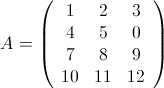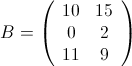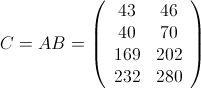## 存储方式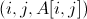1     1     1
1     2     2
1     3     3
2     1     4
2     2     5
3     1     7
3     2     8
3     3     9
4     1     10
4     2     11
4     3     12

1     1     10
1     2     15
2     2     2
3     1     11
3     2     9

## 计算模型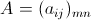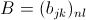，那么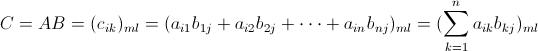（1）在Map阶段，把来自表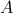的元素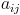，标识成条<key, value>的形式。其中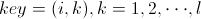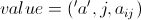；把来自表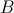的元素，标识成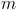条<key, value>形式，其中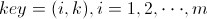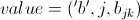（3）在Reduce阶段，有两个问题需要自己问问：
• 当前的<key, list(value)>对是为了计算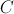的哪个元素？
• list中的每个value是来自表或表的哪个位置？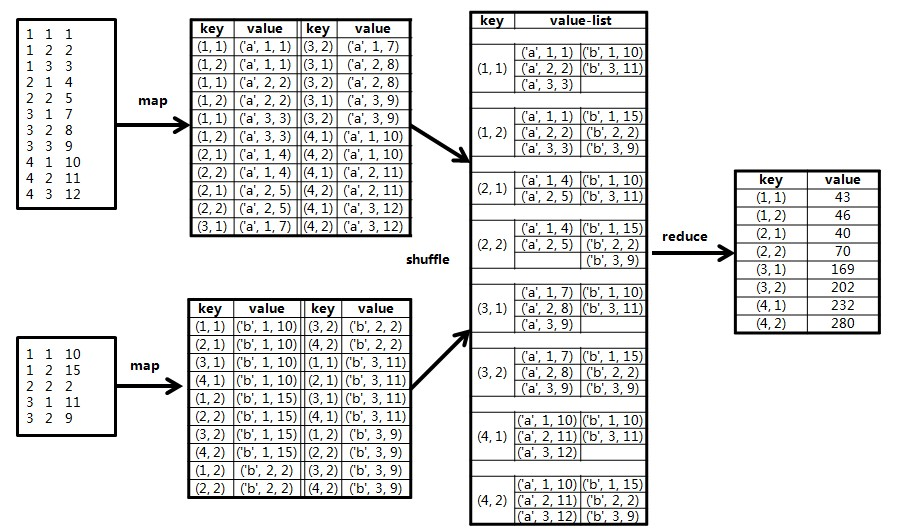## 代码

import java.io.IOException;
import java.util.HashMap;
import java.util.Iterator;
import java.util.StringTokenizer;

public class Bigmmult {
public static final String CONTROL_I = "\u0009";
public static final int MATRIX_I = 4;
public static final int MATRIX_J = 3;
public static final int MATRIX_K = 2;

public static String makeKey(String[] tokens, String separator) {
StringBuffer sb = new StringBuffer();
boolean isFirst = true;
for (String token : tokens) {
if (isFirst)
isFirst = false;
else
sb.append(separator);
sb.append(token);
}
return sb.toString();
}

public static class MapClass extends MapReduceBase implements
Mapper<LongWritable, Text, Text, Text> {
public static HashMap<String , Double> features = new HashMap<String, Double>();

public void configure(JobConf job) {
super.configure(job);
}

public void map(LongWritable key, Text value, OutputCollector<Text, Text> output,
Reporter reporter) throws IOException, ClassCastException {
// 获取输入文件的全路径和名称
String pathName = ((FileSplit)reporter.getInputSplit()).getPath().toString();

if (pathName.contains("m_ys_lab_bigmmult_a")) {
String line = value.toString();

if (line == null || line.equals("")) return;
String[] values = line.split(CONTROL_I);

if (values.length < 3) return;

String rowindex = values;
String colindex = values;
String elevalue = values;

for (int i = 1; i <= MATRIX_K; i ++) {
output.collect(new Text(rowindex + CONTROL_I + i), new Text("a#"+colindex+"#"+elevalue));
}
}

if (pathName.contains("m_ys_lab_bigmmult_b")) {
String line = value.toString();
if (line == null || line.equals("")) return;
String[] values = line.split(CONTROL_I);

if (values.length < 3) return;

String rowindex = values;
String colindex = values;
String elevalue = values;

for (int i = 1; i <= MATRIX_I; i ++) {
output.collect(new Text(i + CONTROL_I + colindex), new Text("b#"+rowindex+"#"+elevalue));
}
}
}
}

public static class Reduce extends MapReduceBase
implements Reducer<Text, Text, Text, Text> {
public void reduce(Text key, Iterator<Text> values,
OutputCollector<Text, Text> output, Reporter reporter)
throws IOException {

int[] valA = new int[MATRIX_J];
int[] valB = new int[MATRIX_J];

int i;
for (i = 0; i < MATRIX_J; i ++) {
valA[i] = 0;
valB[i] = 0;
}

while (values.hasNext()) {
String value = values.next().toString();
if (value.startsWith("a#")) {
StringTokenizer token = new StringTokenizer(value, "#");
String[] temp = new String;
int k = 0;
while(token.hasMoreTokens()) {
temp[k] = token.nextToken();
k++;
}

valA[Integer.parseInt(temp)-1] = Integer.parseInt(temp);
} else if (value.startsWith("b#")) {
StringTokenizer token = new StringTokenizer(value, "#");
String[] temp = new String;
int k = 0;
while(token.hasMoreTokens()) {
temp[k] = token.nextToken();
k++;
}

valB[Integer.parseInt(temp)-1] = Integer.parseInt(temp);
}
}

int result = 0;
for (i = 0; i < MATRIX_J; i ++) {
result += valA[i] * valB[i];
}

output.collect(key, new Text(Integer.toString(result)));
}
}
}

## 参考资料

02-175万+
11-30111
01-05497
04-171521
09-0345万+
02-169047
02-281479
05-092万+
03-171399
01-15621
10-19434
08-15825
09-13245
08-116万+
08-141万+
03-1258
03-231150

### “相关推荐”对你有帮助么？

•非常没帮助
•没帮助
•一般
•有帮助
•非常有帮助被折叠的  条评论 为什么被折叠?到【灌水乐园】发言Chungtow-Leo

¥2 ¥4 ¥6 ¥10 ¥20余额支付 (余额：-- )扫码支付获取中扫码支付点击重新获取扫码支付1.余额是钱包充值的虚拟货币，按照1:1的比例进行支付金额的抵扣。
2.余额无法直接购买下载，可以购买VIP、C币套餐、付费专栏及课程。余额充值